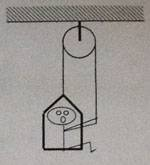# One-dimensional dynamics question

Ascendant78

## Homework Statement

A 60kg student lowers herself down 40m at a constant speed of 1.0 m/s. The chair has a mass of 35kg. Find the force of the rope. (See image)## Homework Equations

I was using force equations in attempts to solve for the problem.

## The Attempt at a Solution

Fchair+Fstudent-Frope-Frope=m(a)
35(9.8)+60(9.8)-2Frope=95(0)
343+588-2Frope=0
2Frope=931
Frope=465.5N

Although the answer I got above was 465.5N, the answer listed in the back of the book was Frope=490N. I am at a complete loss as to what I am doing wrong here? I did a similar problem where the person was traveling upwards like this at a constant velocity and got it right. Not sure what changed here that I am not taking into consideration?

I am also curious after having done a similar problem, since the force is split into 2 parts, the force quantity for the rope would be the value for each rope individually, correct (one attached to chair and the one held by the student)? I just want to make sure I am understanding this correctly. Also, if that is the case, then if I were asked what the total force being applied to the rope was, it would be double the value to account for each side being pulled, correct?

Last edited:

Your solution looks fine to me. Could be the student in question lost 5kg in the time between editions of the bookYour solution looks fine to me. Could be the student in question lost 5kg in the time between editions of the book